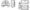# CSVTU Exam Papers – BE I Year – Engineering Graphics– 2005

BE (1st year)

Examination – 2005

Engineering Graphics

(a) Construct a scale of 1.5 to show decimeters and centimeters and to read up to 1 m. Show a length of 7.6 dm on it.

(b) A ball thrown op in the air reaches a maximum height of 45 meters and travels a horizontal distance of 75 meters. Trace the path of the ball, as summing it to be parabolic.

or

(a) Construct a diagonal scale of R.F.= 1/4000 to show meters and long enough the measure up to 500 meters.

(b) Show by means of a drawing that when the diameter of directing circle is twice that of generating circle, the hypocycloid is a straight line. Take the diameter of the generating circle equal to 50 mm.

A line PQ 100 mm long, is inclined at 30° to HP and at 45° to VP. Its midpoint is in the VP and 20 mm above the HP. Draw it projections, if its end p is in the third quadrant and Q in the first quadrant.

or

The top view of a 75 mm long line AB measures 65 mm while the length of its front view is 50 mm. Its one A is In the HP and 12 mm in front of V.P Draw the projections of AB and determine the inclinations with the H.P. and V.P.

A hexagonal pyramid, base 25 mm side and axis 50 mm long, has an edge of its base on the ground. Its axis is inclined at 30° to the ground and parallel to the V.P. Draw its projections.

or

A hexagonal pyramid, base 25 mm side axis 55 mm long, has of its base edge on the ground. A plane containing that edge and axis is perpendicular to the ground and inclined at 45 to VP Draw its projection when the apex is nearer the VP than the base.

(a) Draw the development of the lateral surface of the frustum of the square pyramid.

(b) Draw the isometric view of the any of the following two blocks, views of which are named as (A) and (B) bellowPart (a) is compulsory Solve one part from (b) or (c)

(a) What if meaning of following commands/ tools in auto CAC Mirror, Rotate, TRim.

(b) Write steps with commands to dimension arcs and circles.

(c)  Write steps with commands to create horizontal Dimension using dimension tool bar.